## 5.11 This one seems easy.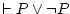Let's see ifis as easy as some say: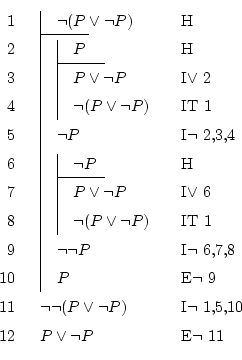One of the simplest but longest I found. It seems even unnecessary to prove this, since everyone knows that between today it's Thursday'' and today it's not Thursday'', one of them is true (they can't be both be false at the same time).

We could start by thinking in the proof by cases method, since from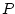we can extract, and from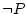we can extract, so, the same formula. But this doesn't help, since proof by cases is the disjunction elimination, and we don't have any disjunction to eliminate; in fact, we also don't have the truth formula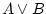in which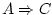and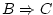, as the rule needs. Actually, we don't have any formula which we know it's true (as the left part of the sequent is empty).

We know that we must start with a hypothesis (there's no alternative). Since it's rather clear thatis true, it may also be easy to prove that its contrary,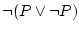, is false. So we will use reduction to the absurd: doing that supposition on line 1, we must achieve a contradiction, any one.

I proposed myself to achieve the contradictionand. But we don't have any of these formulas; how can we obtain them? Doing reduction to the absurd again is an option: to see that, suppose thatand get a contradiction. As we did in another occasions, it's very useful to profit the capabilities of the disjunction introduction: having supposed, we can convert it toto search our contradiction. As we have theat the top, we can use it to finish by demonstrating. We can do the same to prove, but this time supposing.

Having obtainedandafter supposing, we see that this formula can't be true, so its negation,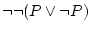, is. By negation elimination, we get our searched formula:.

I did it this way to make it rather symmetrical, but it can be shorter if we search another contradiction, for instanceand. Then it would be like this: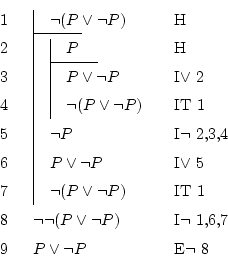Daniel Clemente Laboreo 2005-05-17